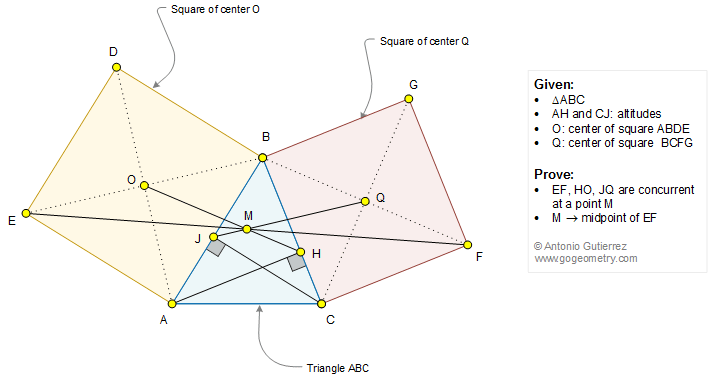Geometry Problem 1293

Elements: Triangle, Altitudes, Two Squares, Center, Concurrent Lines, Midpoint

The figure below shows a triangle ABC with the altitudes AH and CJ. O and Q are the centers of the squares ABDE and BCFG, respectively. Prove that (1) EF, HO, and JQ are concurrent at a point M; (2) M is the midpoint of EF.Geometric Art of Problem 1293: Sketching, Typography, iPad Apps